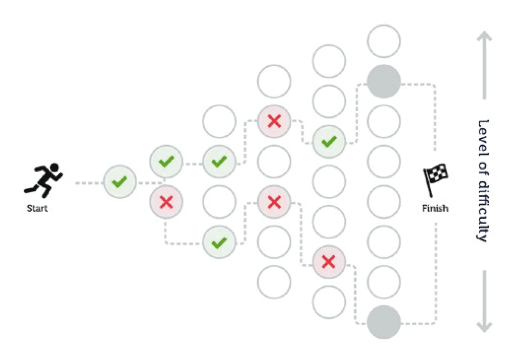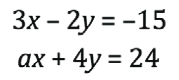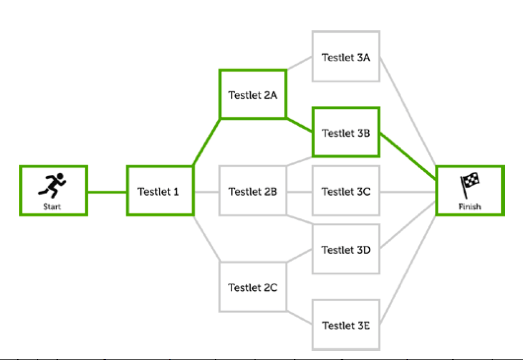# Two Types of Computer Adaptive TestingLet’s look at two types of adaptive testing. The first type, item adaptive, adjusts each future item based on an average estimated ability, called the ability estimate. This type of adaptive testing has many possible uses. For example, an ideal use for this technology is vocabulary testing, where students are presented with words and asked to define them (or they are presented definitions and asked for the correct term). When a student answers incorrectly, the student is presented with lower-level vocabulary terms. As students get questions correct, the terms and/ or definitions can become increasingly difficult and complex.Image courtesy of Learnosity

Another potential use is in mathematics. An instructor can select a specific topic or standard, such as solving a system of linear equations. Questions at a lower level of difficulty might consist of equations already set up to be solved using substitution and make use of nice, evenly divisible integers. Questions at a mid-range level difficulty might require students to manipulate the given equations before they can be solved using substitution or another method. These questions might also include fractions or decimals. Finally, questions at a high level of difficulty might model the one below:If the solution to the system of equations above is (–1, 6), what is the value of a?

This type of adaptive model can be used with any question type, across any discipline. Literature and social science questions can require an increasing level of understanding, starting with recite-level questions and progressing to analyze-level questions. Science questions can begin at the recall level and advance to complex application questions. Instructors can also make the tests broad in nature, covering a wide range of topics and varying levels of difficulty, or test specific areas of knowledge, like the first examples given. In either case, the applications of the adaptive assessment are both wide and far-reaching.

A second type of adaptive assessment offered by Learnosity is called branching. This method of adaptive testing allows item creators to form mini-tests, called testlets, which consist of groups of questions or activities to create larger chunks of questions, which are subject to the adaptive algorithm. In this scenario, each testlet is chosen based on the student’s ability estimate up to that point. This approach allows teachers to progressively test topics rather than individual questions.Image courtesy of Learnosity

For example, a teacher might begin with a question set on adding and subtracting integers. This could progress to a group of questions that assess adding and subtracting decimals. If answered correctly, the next testlet might consist of multiplying and dividing integers. Finally, the highest-level testlet might be made of questions related to multiplying and dividing decimals. In this way, teachers are assessing slightly different skill sets in a related umbrella subject matter.

In the humanities, testlets might begin at the recall, identify, and define levels. A student might move on to a testlet consisting of classify, categorize, and summarize-level questions. Then, the next testlet might be made up of compare and contrast, assess, and hypothesize questions. Finally, at the highest level, testlets might be composed of critique, analyze, and apply items.

These testlets can each test a specific standard or topic separately, or each testlet can include a variety of topics, set to increasing levels of difficulty. This gives teachers the complete flexibility to focus either on depth or breadth of knowledge, or a combination of both.

Overall, the customization and individualization of the adaptive assessment paradigm allows instructors and school officials to administer more accurate and effective assessments that are both useful to the instructor and relevant to the student.